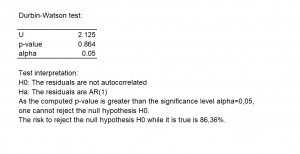# Durbin-Watson test

The Durbin-Watson test checks if there is autocorrelation among the residuals of a linear regression. It is available in Excel using the XLSTAT software.## Durbin Watson test definition

Developed by J.Durbin and G.Watson (1950,1951), the Durbin-Watson test is used to detect the autocorrelation in the residuals from a linear regression.

In practice, the errors are often autocorrelated, it leads to undesirable consequences such as sub-optimal least-squares estimates.

Assume that the error terms (epsilon) are stationary and normally distributed with mean 0. The null and alternative hypotheses of the Durbin-Watson test are:

• H0: The errors are uncorrelated
• H1: The errors are AR(1)

And the test statistic D is:In the context of the Durbin-Watson test, the main problem is the evaluation of the p-values. In XLSTAT the Imhof procedure (1961) is used to solve this problem.

## Options of the Durbin-Watson test in XLSTAT

Significance level (%): Enter the significance level for the test (default value: 5%)

Order: Enter the order, i.e. the number of lags for the residuals (default value: 1)

Do not accept missing data: Activate this option so that XLSTAT does not continue calculations if missing values have been detected.

Remove observations: Activate this option to remove the observations which include missing data.

Replace by the average of the previous and next values: Activate this option to estimate the missing data by the mean of the first preceding non missing value and of the first next non missing value.

Ignore missing data: Activate this option to ignore missing data.

Descriptive statistics: Activate this option to display descriptive statistics for the residuals.

## Results of the Durbin-Watson test in XLSTAT

In XLSTAT, the results of the Durbin-Watson test are the following:

• The tables of descriptive statistics show the simple statistics for the residuals. The number of observations, missing values, the number of non-missing values, the mean and the standard deviation (unbiased) are displayed.

• The value of the statistic D and the p-value of the Durbin-Watson test. A short interpretation is also displayed.

## Example

A tutorial on the Durbin-Watson test is available on our helpcenter.### analyze your data with xlstat

14-day free trial

Included in

Related features